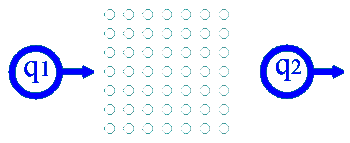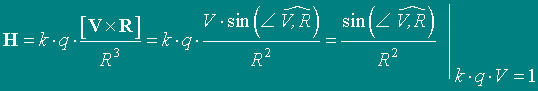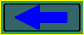﻿ About the possible mechanism of hard radiation in systems of binary stars

The possible mechanism of hard radiation
in systems of binary stars
at presence the interstars accretion channel.

As is marked in article "Equations of Maxwell", there is a magnetic field (MF) between two corpuscles, which move to one party (Fig.8). To be convinced of it, it is enough to calculate intensity of MF in points of a matrix shown in a Fig. 1.Fig. 1

The magnetic intensity of a mobile charged corpuscle can be calculated by formula:The corpuscles in an accretion channel have longitudinal component of motion in basic. Because of rotation of a system of a binary star (Fig. 2), there is, also, a mutual relative motion of corpuscles of interstellar plasma and an accretion channel (Fig. 3). The cross-sectional (I apologize, but, while, in Russian) oscillations of charged particles occur in a channel. The undulatory radiation with a different wavelength and intensity is generated. (�Maxwell's equations" Fig. 11, �Free Electrones Laser�). The radiation is spread in some cone, and the center of a cone of radiation is screened by a star - acceptor. At rotation of such system the double impulses of radiation (Fig. 2) will be watched.Fig. 2Fig. 3